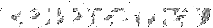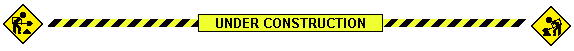COSMIC CONSTANTS
and units via logarithm

The fundamental units used in physics are

• meter (m) for distance (space)
• second (s) for duration (time)
• kilogram (kg) for mass (inertia)
• The choice of these is determined by the nature of the human perception of reality. On the other hand, there are three fundamental "cosmic" constants. Ignore their values and look at the units they are expressed in the table below left:

 [c] = m/s [h] = m2 kg/s [G] = m3 / s2kg [length] = (h G / c3)1/2 [time] = (h G / c5)1/2 [mass] = (h c / G)1/2

This is a well known idea that one can use these constants to construct the "natural" units of length, time and mass. They are called Planck's units (displayed in the table above right). The meaning of the Planck units are not fully understood yet.

How is their existence possible (arithmetically)? Here is my remark: express the cosmic constants in a "logarithmic way," say:

 c = m - s h = 2m + kg - s G = 3m - 2s -kg

Think of these linear equations as a transformation. The matrix of the transformation of units (see the left table below) is nondegenerate, hence its inverse exists and reveals the right powers of the cosmic constants (right table below) when defining the Planck units of length, time and mass.

Constant's matrix
m s kg
c 1 -1 0
h 2 -1 1
G 3 -2 -1

The inverse matrix
c h G
m -3/2 1/2 1/2
s -5/2 1/2 1/2
kg 1/2 1/2 -1/2

You may think of two bases in the same three-dimensional space in which every physical magnitude is represented by a vector of its units (understood---as above---in a "logarithmic way).

 UNIT SPACE to be added soon

Homework: locate basic physical magnitudes as vectors in the Unit Space, such as

 speed =   m - s acceleration =   m - 2s force =   m - 2s + kg pressure =  -m - 2s + kg density =  -3m + kg

Have fun. Add electric field, magnetic field, dielectric constant, current, action, etc.GR space QM 3 time TR 2 -2 mass 1 -1 -1 -1 1 0